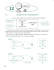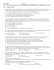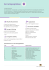# Ch7 Section 3: Confidence Intervals and Sample Size for Proportions

## Transcription

Ch7 Section 3: Confidence Intervals and Sample Size for Proportions
```Ch7 Section 3: Confidence Intervals and Sample Size for
Proportions
Belief in Haunted Places
A random sample of 205 college
students was asked if they believed
that places could be haunted, and
65 of them responded yes. In this
section we’ll learn how to estimate
the true proportion of college
students who believe in the
possibility of haunted places.
According to Time magazine, 37%
of Americans believe that places
can be haunted. Source: Time
magazine, Oct. 2006.
CH7: Confidence Intervals and Sample Size
Santorico - Page 242
TERMINOLOGY and DETAILS:
The population proportion will be denoted by the letter p.
The point estimate of the population proportion is the sample
proportion.
ˆ , called p hat.
We will symbolize the sample proportion by p
For large random samples, the central limit theorem tells us
that the sampling distribution of the sample proportion is
approximately normal.

The distribution will have mean p and a standard deviation of
pq /n.
CH7: Confidence Intervals and Sample Size

Santorico - Page 243
The central limit theorem tells us what?!?
If we take a bunch of samples they
will have proportions that fall
around the true population
proportion.
AND, the sample proportions will
have a distribution that looks
normal over the samples.
We can use the same ideas as in Section 7-1 to construct a
confidence interval for the population proportion p.
CH7: Confidence Intervals and Sample Size
Santorico - Page 244
Let’s look at a simulation experiment to reinforce this concept:
 The main website for the simulation is
http://socr.ucla.edu/htmls/SOCR_Experiments.html
 You will want to select “Binomial Coin Experiment” in the
drop down menu on the right
 Here the coin represents the binomial event that
corresponds to the proportion.
 You can change the sample size, n, and the population, p.
 And, create samples!
 Try different combinations and see what the sampling
distribution for the sample proportion looks like.
 This is the Central Limit Theorem!
CH7: Confidence Intervals and Sample Size
Santorico - Page 245
Formula for a Confidence Interval for a Proportion
Assumptions:
1. The data are a random sample from the population.
ˆ and nqˆ are each greater than or equal to 5.
2. Both np
Formula:


pˆ  z /2
pˆ qˆ
n
Rounding Rule for a confidence interval for a proportion: round to 3
decimal places.
 construct a confidence interval for a population
Concept: Once we
proportion, what’s the probability our interval contains the true
population proportion?
CH7: Confidence Intervals and Sample Size
Santorico - Page 246
Example: In a survey of 935 Denver residents, 60% said that
they believed in aliens. Calculate a 95% confidence interval for
the proportion of Denver residents who believe in aliens.
pˆ  0.6
qˆ  0.4
n  935
z /2  1.96
Check assumptions first!

 that the 935 represent a random sample
 1. We must assume
of Denver residents.
2. Since npˆ  935  0.6  561 and nqˆ  935  0.4  374 , we
have satisfied our second requirement that these be 5.
CH7: Confidence Intervals and Sample Size
Santorico - Page 247
Next compute the interval:
pˆ  z /2
ˆˆ
pq
0.6  0.4
 0.6  1.96
n
935
 0.6  0.0314019
 (0.569, 0.631)
Finally, interpret: We are 95% confident that the
proportion of Denver residents that believe in aliens is
between 0.569 and 0.631.
CH7: Confidence Intervals and Sample Size
Santorico - Page 248
Back to our motivating example: A random sample of 205
college students was asked if they believed that places could be
haunted, and 65 of them responded yes. Estimate the true
proportion of college students who believe in the possibility of
haunted places with 99% confidence.
pˆ 
qˆ 
n
z /2 
Check assumptions:


CH7: Confidence Intervals and Sample Size

Santorico - Page 249
Compute interval:
pˆ  z /2
pˆ qˆ
n
Interpret!
CH7: Confidence Intervals and Sample Size
Santorico - Page 250
Sample Size for Proportions
Similar to Section 7-1, we can determine the sample size
necessary to achieve the desired precision of a confidence
interval.
Formula for Minimum Sample Size Needed for Interval
Estimate of a Population Proportion:
z /2 2
n  pˆ qˆ  , where E is the desired level of precision. If
 E 
necessary, round up to obtain a whole number.
If an estimate of the proportion isn’t given, use pˆ  0.5 since this
is the worst case scenario (you will have to sample the most
subjects to obtain the desired precision).

CH7: Confidence Intervals and Sample Size
Santorico - Page 251
Example: How large a sample should be surveyed to estimate
the true proportion of college students who do laundry once a
week within 3.5% with 99% confidence? A previous study
placed the proportion around 75%.
2
2
2.575 
 z /2 

ˆ ˆ
n  pq
 0.75  0.25  
  1014.892  1015

 0.035 
 E 
CH7: Confidence Intervals and Sample Size
Santorico - Page 252
Example: A research wishes to estimate the proportion of
executives who own a car phone. She wants to be 90%
confident and be accurate within 5% of the true proportion.
Find the minimum sample size necessary.
z /2 2
n  pˆ qˆ  
 E 
CH7: Confidence Intervals and Sample Size
Santorico - Page 253
```

### Sample size Student Learning Centre Semester 2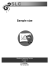### DETERMINING SAMPLE SIZE FOR RESEARCH ACTIVITIES ROBERT V. KREJCIE### measure attention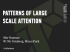### What is a sample proportion?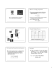### Proportion Power Point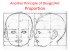### Objectives Qualls - 6. Confidence Interval of Population Proportion Lecture 6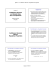### 12 Solving Percents Using Proportions